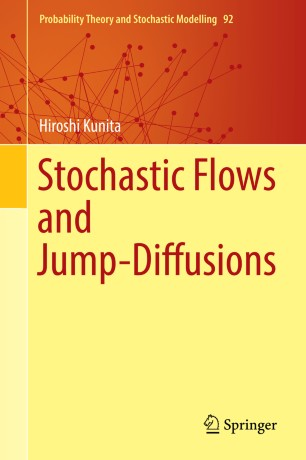Stochastic Flows and Jump-Diffusions

• Hiroshi KunitaPart of the Probability Theory and Stochastic Modelling book series (PTSM, volume 92)

1. Front Matter
Pages i-xvii
2. Hiroshi Kunita
Pages 1-44
3. Hiroshi Kunita
Pages 45-75
4. Hiroshi Kunita
Pages 77-124
5. Hiroshi Kunita
Pages 125-166
6. Hiroshi Kunita
Pages 167-244
7. Hiroshi Kunita
Pages 245-302
8. Hiroshi Kunita
Pages 303-340
9. Back Matter
Pages 341-352

Introduction

This monograph presents a modern treatment of (1) stochastic differential equations and (2) diffusion and jump-diffusion processes. The simultaneous treatment of diffusion processes and jump processes in this book is unique: Each chapter starts from continuous processes and then proceeds to processes with jumps.
In the first part of the book, it is shown that solutions of stochastic differential equations define stochastic flows of diffeomorphisms. Then, the relation between stochastic flows and heat equations is discussed. The latter part investigates fundamental solutions of these heat equations (heat kernels) through the study of the Malliavin calculus. The author obtains smooth densities for transition functions of various types of diffusions and jump-diffusions and shows that these density functions are fundamental solutions for various types of heat equations and backward heat equations. Thus, in this book fundamental solutions for heat equations and backward heat equations are constructed independently of the theory of partial differential equations.
Researchers and graduate student in probability theory will find this book very useful.

Keywords

stochastic differential equation with jumps jump-diffusion process Malliavin calculus Wiener space fundamental solution asymptotic short time estimate smooth density stochastic flow diffeomorphism diffusion and jump-diffusion processes heat equations backward heat equations 60H05, 60H07, 60H30 35K08, 35K10, 58J05

Authors and affiliations

• Hiroshi Kunita
• 1
1. 1.Kyushu University (emeritus)FukuokaJapan

Bibliographic information

• DOI https://doi.org/10.1007/978-981-13-3801-4
• Copyright Information Springer Nature Singapore Pte Ltd. 2019
• Publisher Name Springer, Singapore
• eBook Packages Mathematics and Statistics
• Print ISBN 978-981-13-3800-7
• Online ISBN 978-981-13-3801-4
• Series Print ISSN 2199-3130
• Series Online ISSN 2199-3149
• Buy this book on publisher's site